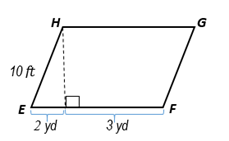Chapter 8.CT, Problem 5CT### Elementary Geometry for College St...

6th Edition
Daniel C. Alexander + 1 other
ISBN: 9781285195698

#### Solutions

Chapter
Section### Elementary Geometry for College St...

6th Edition
Daniel C. Alexander + 1 other
ISBN: 9781285195698
Textbook Problem
1 views

# In square feet, find the area of ▱ E F G H .                                   _To determine

To find:

The area of EFGH in feet.

Explanation

Given:

The figure given below.

Theorem:

1. The area A of a parallelogram whose base has length b and with corresponding altitude of length h is given by A=bh.

2. 1 yard = 3 feet

From the given figure, EF=b=5 yd or b=15 ft, EI=2 yd or 6 ft, and let the altitude be HI=h.

In the right triangle EHI,

HI2+EI2=HE2

h2+62=102

h2=102-62

<

### Still sussing out bartleby?

Check out a sample textbook solution.

See a sample solution

#### The Solution to Your Study Problems

Bartleby provides explanations to thousands of textbook problems written by our experts, many with advanced degrees!

Get Started

#### g(x,y)=xy

Calculus: Early Transcendental Functions (MindTap Course List)

#### Evaluate the integral. 8. dtt2t216

Single Variable Calculus: Early Transcendentals

#### 13.

Mathematical Applications for the Management, Life, and Social Sciences

#### Graph each equation. x+y57=3x

College Algebra (MindTap Course List)